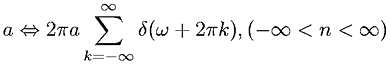Equations > Signal Processing > Fourier Transform Pairs > Discrete-Time Fourier transform of a constant

### Discrete-Time Fourier transform of a constantLatex Code:

MathML Code:

 $a⇔2\pi a\sum _{k=-\infty }^{\infty }\delta \left(\omega +2\pi k\right),\left(-\infty

MathType 5.0: